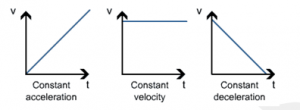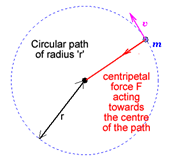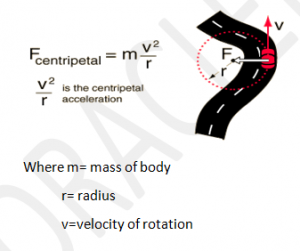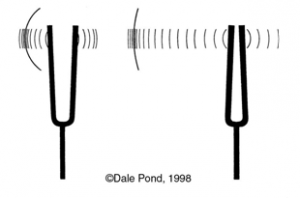# UKPCS Science: Physics- Motions – Units and Measurements #3

Oracle IAS, the best coaching institute for UPSC/IAS/PCS preparation in Dehradun brings to you Newton Laws of Motion UKPCS Science (paper #6).-

Motions

Linear motion (also called rectilinear motion is a motion along a straight line, and can therefore be described mathematically using only one spatial dimension. The linear motion can be of two types: uniform linear motion with constant velocity or zero acceleration; non uniform linear motion with variable velocity or non-zero acceleration. The motion of a particle (a point-like object) along a line can be described by its position x, which varies with t (time). An example of linear motion is an athlete running 100m along a straight track. Newton Laws of MotionLinear motion is the most basic of all motion. According to Newton’s first law of motion, objects that do not experience any net force will continue to move in a straight line with a constant velocity until they are subjected to a net force. Under everyday circumstances, external forces such as gravity and friction can cause an object to change the direction of its motion, so that its motion cannot be described as linear.

Circular motion:

In physics, circular motion is a movement of an object along the circumference of a circle or rotation along a circular path. It can be uniform, with constant angular rate of rotation and constant speed, or non-uniform with a changing rate of rotation. Examples of circular motion include: an artificial satellite orbiting the Earth at constant height, a stone which is tied to a rope and is being swung in circles.Since the object’s velocity vector is constantly changing direction, the moving object is undergoing acceleration by a centripetal force in the direction of the center of rotation. Without this acceleration, the object would move in a straight line, according to Newton’s laws of motion.Vibratory motion

A type of motion in which a particle moves to and fro about a fixed point.It is quite similar to oscillations. Actually it is the same as oscillations. .The only difference is that the term VIBRATION is used when oscillations are having high frequency, or in simple terms, when the particle moves very fast about a fixed point. Example vibration of tuning fork.

Cost- Rs.5000/- (including shipping)

(~2500+ pages)

# UKPCS Mains Study Material subject wise

The notes are strictly as per UKPCS syllabus (topic wise):

Individual Polity Cost: Rs. 1500/- (including shipping)

Individual S&T Cost: Rs. 1500/- (including shipping)

Individual Geography Cost: Rs. 1500/- (including shipping)

Individual Economics Cost: Rs. 1000/- (including shipping)